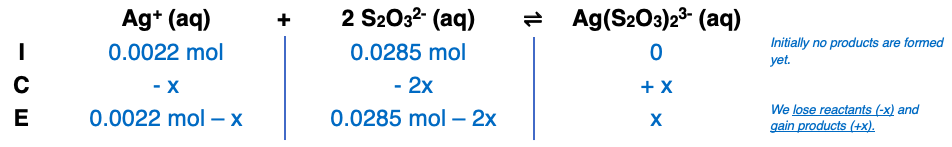# Problem: What is [Ag+] when 25.0 mL each of 0.044 M AgNO3 and 0.57 M Na2S2O3 are mixed? [Kf of Ag (S2O3)2 3− = 4.7×1013]?

###### FREE Expert Solution

Step 1:

Total volume = 25.0 mL + 25.0 mL = 50.0 mL

[Ag+] = 0.0022 M Ag+

[S2O32-] = 0.0285 M S2O32-

Step 2:###### Problem Details

What is [Ag+] when 25.0 mL each of 0.044 M AgNO3 and 0.57 M Na2S2O3 are mixed? [Kf of Ag (S2O3)2 3− = 4.7×1013]?